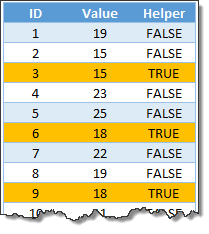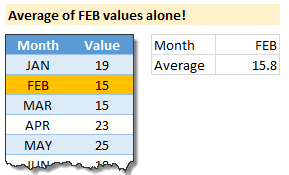# Calculating average of every nth value [Formula tips]

Share

Lets say you have a large list of numbers, and you want to calculate the average of every nth value. Not the average of all numbers, but just every nth number.

That is what we will learn in next few minutes.

### Few assumptions

Before we jump in to any formulas, first lets assume that all your data is in a table, conveniently named as tbl. Lets say this table has below structure.Also, the value of n is a named cell N.

## Average of every nth value

### Approach 1: Using helper columnsIf you have no allergies towards nuts, dairy or helper columns, then this approach is easiest.

We just add an extra column to our tbl , called as helper.

In the helper column, write this formula.

=MOD([@ID], N)=0

This will fill the helper column with TRUE & FALSE values, TRUE for all nth values, FALSE for everything else. See aside.

Once we have the helper column, calculating average of every nth value is easy as eating every slice of a cake.

We use AVERAGEIF to do this.

=AVERAGEIF(tbl[Value],tbl[Helper],TRUE)

### Approach 2: Not using helper columns

Now things get interesting. Lets say you want to calculate average, but not use any helper columns.

First the formula:

=AVERAGE(IF(MOD(tbl[ID], N)=0,tbl[Value]))

Array entered.

Lets understand how it works:

We want the average of every nth item of tbl[Value] column.

In other words, we want average of every item of tbl[Value] column, whose corresponding tbl[ID] value is perfectly divisible by n.

How do we know when a value is perfectly divisible by another?

Don’t worry. You don’t have to do the long division on paper now. Instead we use Excel’s MOD function.

When a value is perfectly divisible by another, the reminder is zero.

So, MOD(value1, value2) = 0 means, value2 divides value1 perfectly.

That means…

We want the average of tbl[Value] when MOD(tbl[ID], N) = 0

Lets write that in Excel formula lingo.

=AVERAGE( IF(MOD(tbl[ID], N) = 0, tbl[Value]) )

This formula results in a bunch of values and FALSEs. Assuming N=3, this is what we get (for sample data):

=AVERAGE({FALSE;FALSE;15;FALSE;FALSE;18;FALSE;FALSE;18;FALSE;FALSE;15;FALSE;FALSE;14; …})

Since AVERAGE formula ignores any logical values, it will calculate the average of {15, 18, 18, 15, 14 … } and returns the answer you are expecting.

As this formula is processing arrays instead of single values, you need to array enter it (CTRL+SHIFT+Enter after typing the formula).

### Bonus scenario: Average of FEBRUARY values only!

Here is a bonus scenario. Lets say you want to calculate the average sales of FEB alone… Then you can use AVERAGEIF (or AVERAGEIFS, if you want to have multiple conditions).

=AVERAGEIF(tbl[value], tbl[month], “FEB”)Click here to download the example workbook. It contains all the techniques explained in this post. Play with the data & formulas to understand better.

### Time for some challenges…

If you think averaging every nth value is not mean enough, try below challenges. Post your answers using comments.

1. Write a formula to calculate average of every nth value, starting at row number ‘t’.
2. Write a formula to calculate average of every nth value, assuming your table has only value column (no ID column).

### Improving your Excel batting average

Calculating averages predates slice bread. Folklore says that when first neanderthal figured out how to express numbers and carved 2 of them on a cave wall, his manager walked by and asked “What is the average of these two? Eh?” and thumped her chest.

Although caves & wall carvings are replaced by cubicles & spreadsheets, we are still calculating averages, almost 2.9 million of them per hour.

So it pays to learn a few tricks about Excel Average formulas. Check out below to improve your average:

If your boss is the kind who thumps her chest and mocks you for your poor Excel skills, don’t cave in. Fight back. Enroll in Excel School and show that you can evolve.

### Get FREE Excel + Power BI Tips

Simple, fun and useful emails, once per week.

Learn & be awesome.

### Welcome to Chandoo.org

Thank you so much for visiting. My aim is to make you awesome in Excel & Power BI. I do this by sharing videos, tips, examples and downloads on this website. There are more than 1,000 pages with all things Excel, Power BI, Dashboards & VBA here. Go ahead and spend few minutes to be AWESOME.

Read my storyFREE Excel tips book

I will be presenting at two events in London in April 2020. Come & join me.

Chandoo is an awesome teacher
5/5

– Jason

Still on fence about Power BI? In this getting started guide, learn what is Power BI, how to get it and how to create your first report from scratch.

## Related Tips

### 16 Responses to “Calculating average of every nth value [Formula tips]”

1.JLeno says:

I think the helper column can be replaced by a ROW function:
(array entered)

and including every nth value after row t (specified in cell H5):
(array entered)

Don't know if there's a shorter version I'm missing here 🙂

2.MF says:

=AVERAGE(IF(MOD((ROW(tbl)-ROW(tbl_start)),N)=0,tbl))
Array Enter

assume tbl has a header, and "tbl_start" refers to the cell of the header

3.JD Hen says:

"If you think averaging every nth value is not 'mean' enough" 🙂

4.Andrew says:

Here's a non array formula version that can be used with any range of values and any value for N:

=SUMPRODUCT(--(MOD(ROW(ValRange)-CELL("row",ValRange)+1,N)=0),ValRange)/INT(ROWS(ValRange)/N)

I'm sure there's a way to shorten this, but just my first attempt!

5.Sumit Bansal says:

I have combined both part (starting at row number T and not using helper column) [G2 has the value of T]

=IFERROR(SUMPRODUCT(INDEX(tbl[Value],G4):INDEX(tbl[Value],COUNT(tbl[Value]))*(MOD(ROW(INDIRECT("1:"&COUNT(tbl[Value])-G4+1)),G5)=0))/INT((COUNT(tbl[Value])-G4)/20), "Out of list Range")

6.Legei says:

Hi Chandoo, I want to know, why the formula "averageifs" can't be replaced by "averageif". It seems that there is just one criteria required to the calculation, no need to use the formula "averageifs".

•Chandoo says:

They both work Legei. I have used AVERAGEIFS as it will let you add more conditions if needed.

•PSG says:

Hi Chandoo,

Strangely Approach 1: AVERAGEIF(tbl[Value],tbl[Helper],TRUE) is not working for me as well.

Whereas if you use AVERAGEIFS, the function is working fine. Can you please double check if it (AVERAGEIF) works for you?

Thanks!!
PSG

•Andrew says:

Use AVERAGEIF(tbl[Helper],TRUE,tbl[Value])

7. […] Calculating average of every nth value […]

8.Trevor says:

How might one perform this function with the following restrictions:
1) the table has no ID column, rather just a single column of values, and
2) the table does not start conveniently at cell A1, but rather in a random place on the worksheet?

9.Marvin says:

I don't understand the [@id] notation, can you point me to something that clarifies?

10.Moom says:

When I recalculate the excel file for the*=AVERAGE(IF(MOD(tbl[ID],\$G\$5)=0,tbl[Value]))*, the value become zero, and the evaluation of the formula become AVERAGE(IF(MOD(1,20)=0,tbl[value])).
And further evaluation give AVERAGE(IF[FALSE,tbl[value]).
I really don't know how to fix this problem.
P.S I am using EXCEL 2010

11.Mithun Pandey says:

+AVERAGE(MOD(ROW(INDIRECT("1:"&COUNT(a_list))),3)=0,a_list)

with ctrl + shift + enter

I think this works ?

12.Mithun Pandey says:

+AVERAGE(MOD(ROW(INDIRECT(“1:”&COUNT(a_list))),n)=0,a_list)

i had checked my formula with 3 and hence put 3 in my previous post

13.saleem says:

in colum A i have 1000(values) rows i want to take an average of every 10 values (in colum) . so that it will give me 100 values in colum b. can any Body tell me how can i do that.
please tell me most simple way to do that.
do i have to define macro for that (hope fully not) i have Excel 2010

### Get FREE Excel & Power-BI Newsletter

One email per week with Excel and Power BI goodness. Join 100,000+ others and get it free.